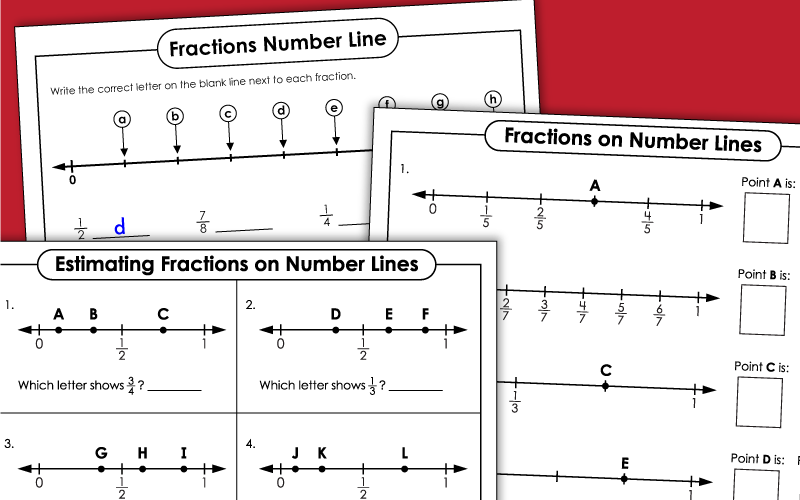# Fractions on Number Lines

Below you'll see a selection of printable worksheets and activities for teaching students to find, identify, and compare fractions on a number line.## Fractions on Number Lines

Determine which fractions are represented by the points on the number lines.
Here's a slightly more challenging worksheet in which students determine the values of the points on the number lines.
Complete each number line by determining the value of the missing points.
This PDF file contains a number line showing equivalent fractions.
Determine the fraction indicated by each lettered point on the number lines.

## Comparing Fractions w/ Number Lines

Match the letters to the fractions on the number line. Then compare fractions using the symbols <, >, and =.
First identify each mixed number on the line. Then compare the mixed numbers.
This double number line contains both fractions and percentages. Use the symbols <, >, and = to compare values.
More Fraction Worksheets

This page has links to different fraction topics on our website, including operations with fractions, comparing and ordering, mixed numbers, reciprocals, and more.

My Account
Site Information